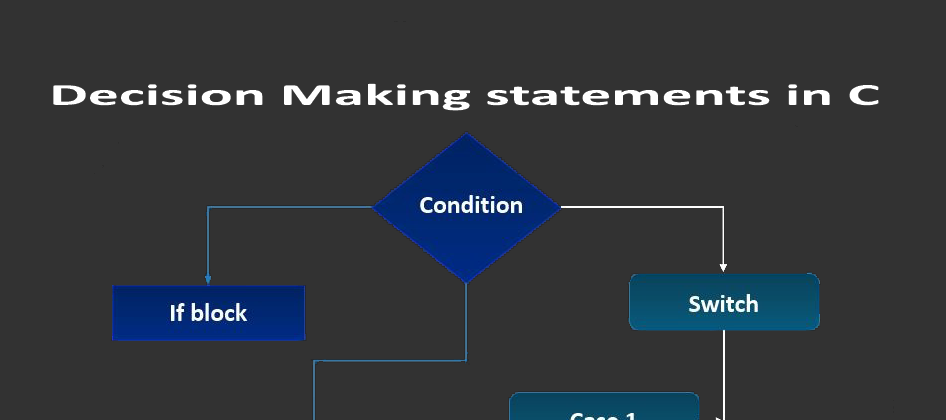## CodeNewbie Community 🌱# Decision Making statements in C

In this tutorial, we will study decision making statements in c, the if statement, else statement, and the if-else statement.

## What are the decision making statements?

Decision Making can be understood by using an example, the age of the student is stored in two-variables but if we want to print the age of the student we can use the decision-making statements (if, if-else, else if, Switch) in this situation.

## What are if statements?

The If statement uses the curly braces { } for defining the block. If the condition is true then the statement inside the block is executed. And if the condition is false then the compiler skips the statements inside the block. The if statement can be used as many times as we want to.

``````if(condition)
{
/* Statements */
}
``````

## What are the if-else statements?

It is similar to the if statement but in this, the else block is added and the else is executed when the condition in the if is false. The If-Else Statement defines right and wrong. Just like the if statement it can be used as many times as we want to.

``````if(condition)
{
/* Statements1*/
}
else
{
/* Statements2*/
}
``````

## What are the else statements?

If in the if and else we want to add a new condition then we have to define the else if block. Unlike the if and if-else statement, we can not use it else as many times as we want to. There can be only one else statement for each if statements.

``````if (condition)
{
/* Statements1*/
}
else if (condition)
{
/* Statements2*/
}
else (condition)
{
/* Statements3*/
}
``````

## What is the switch statement?

The switch statement is similar to the if statement but in the switch statement we check the case. In the switch case, a variable is tested for equality against a list of values. On reaching a particular condition only the statements are executed which are written inside the case. The Case is matched with the integer variable. If the case integer variable is matched then the case is executed.

``````switch (expression)
{
case constant: statement1;
break;
case constant: statement2;
break;
case constant: statement3;
break;
default: statement;
break;
}
``````